# 论文解读（SimGRACE）《SimGRACE: A Simple Framework for Graph Contrastive Learning without Data Augmentation》

2022-05-10 21:53 218 查看

# 1 Introduction

对比学习种数据增强存在的三个问题：

• [li]First, the augmentations can be manually picked per dataset by trial-and-errors.
• Second, the augmentations can be selected via cumbersome search.
• Third, the augmentations can be obtained with expensive domain knowledge as guidance.
[/li]

框架对比：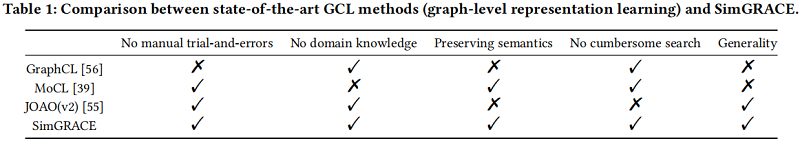# 2 Method

框架如下：## 2.1 SimGRACE

### 2.1.1 Encoder perturbation

GNN encoder $f(\cdot ; \theta)$ 及其扰动版本 $f\left(\cdot ; \boldsymbol{\theta}^{\prime}\right)$ 从同一个图获得其表示：

$\begin{array}{l} \mathbf{h}=f(\mathcal{G} ; \boldsymbol{\theta})\\\mathbf{h}^{\prime}=f\left(\mathcal{G} ; \boldsymbol{\theta}^{\prime}\right)\end{array} \quad\quad\quad(1)$

扰动版本的编码器 $f(\cdot ; \boldsymbol{\theta})$ 的超参数：

$\boldsymbol{\theta}_{l}^{\prime}=\theta_{l}+\eta \cdot \Delta \theta_{l} \quad\text{with} \quad \Delta \theta_{l} \sim \mathcal{N}\left(0, \sigma_{l}^{2}\right) \quad\quad\quad(2)$

其中：

• [li]$\theta_{l}$ 和 $\theta_{l}^{\prime}$ 分别对应着 GNN encoder 及其扰动版本第 $l$ 层的权重参数；
• $\eta$ 代表着扰动大小的系数；
• $\Delta \theta_{l}$ 是从零均值和方差为 $\sigma_{l}^{2}$的高斯分布中采样的扰动项；
[/li]

请注意，BGRL 和 MERIT 在训练期间使用 online encoder 更新 target network 。SimGRACE 与它们的不同之处在于：

• [li]SimGRACE 用随机高斯噪声扰动编码器，而不是动量更新；
• SimGRACE不需要数据增强，而 BGRL和 MERIT 将它作为先决条件；
• SimGRACE专注于图级表示学习，而 BGRL 和 MERIT 只适用于节点级任务；
[/li]

使用非线性投影头 $g(\cdot)$ 提高表示质量：

$\begin{array}{l} z=g(\mathbf{h})\\z^{\prime}=g\left(\mathbf{h}^{\prime}\right)\end{array} \quad\quad\quad(3)$

### 2.1.3 Contrastive loss

在 SimGRACE 训练过程中，随机抽取 $N$ 个图，然后将它们输入GNN编码器 $f(\cdot ; \theta)$ 及其扰动版本 $f\left(\cdot ; \boldsymbol{\theta}^{\prime}\right)$，每个图有两个表示，总共有 $2N$ 个图表示。我们将 minibatch 中的第 $n$ 个图的图表示 $z$ 、$z^{\prime}$ 重新定义为 $z_{n}$ 、$z_{n}^{\prime}$ 。负对是由 minibatch 中的其他 $N−1$ 个图的扰动表示生成的。将余弦相似度函数表示为 $\operatorname{sim}\left(z, z^{\prime}\right)=z^{\top} z^{\prime} /\|z\|\left\|z^{\prime}\right\|$，第 $n$ 个图的对比损失定义为：

${\large \ell_{n}=-\log \frac{\left.\exp \left(\operatorname{sim}\left(z_{n}, z_{n}^{\prime}\right)\right) / \tau\right)}{\sum_{n^{\prime}=1, n^{\prime} \neq n}^{N} \exp \left(\operatorname{sim}\left(z_{n}, z_{n^{\prime}}\right) / \tau\right)}} \quad\quad\quad(4)$

## 2.2 Why can SimGRACE work well?

根据  分析对比学习的表示 质量。其 alignment metric 被直接定义为正对之间的预期距离：

\ell_{\text {align }}(f ; \alpha) \triangleq \underset{(x, y) \sim p_{\text {pos }}}{\mathbb{E}}\left[\|f(x)-f(y)\|_{2}^{\alpha}\right], \quad \alpha>0 \quad\quad\quad(5)

其中 $p_{\text {pos }}$ 是正对的分布（对同一样本的数据增强）。这个度量与对比学习的目标很好地一致：正样本应该在嵌入空间中保持接近。

类似地，对于 SimGRACE 框架，提供了一个修改的 alignment 度量：

\ell_{\text {align }}(f ; \alpha) \triangleq \underset{x \sim p_{\text {data }}}{\mathbb{E}}\left[\left\|f(x ; \boldsymbol{\theta})-f\left(x ; \boldsymbol{\theta}^{\prime}\right)\right\|_{2}^{\alpha}\right], \quad \alpha>0 \quad\quad\quad(6)

其中，$p_{\text {data }}$ 代表着数据分布，本文在实验中设置 $\alpha=2$ 。

另一种是 uniformity 度量，它被定义为平均成对高斯势的对数：

${\large \ell_{\text {uniform }}(f ; \alpha) \triangleq \log \underset{x, y^{i . i \cdot d .}{ }_{\sim} p_{\text {data }}}{\mathbb{E}}\left[e^{-t\|f(x ; \theta)-f(y ; \theta)\|_{2}^{2}}\right] . \quad t>0} \quad\quad\quad(7)$

本文设置 $t=2$ 。uniformity 度量也与对比学习的目标相一致，即随机样本的嵌入应该分散在超球面上。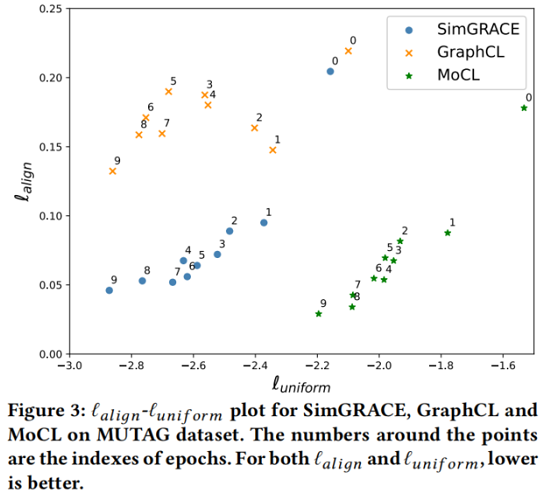在训练过程中，我们每 2 个 epoch 设置一次 SimGRACE、GraphCL 和 MoCL 的检查点，并在 Figure 3 中可视化 alignment \ell_{\text {align }} 和 uniformity $\ell_{\text {uniform }}$。可以观察到，这三种方法都可以改善 alignment 和 uniformity。然而，GraphCL 在 alignment 上比 SimGRACE 和 MoCL 获得的增益更小，换句话说，正对不能在 GraphCL 中保持接近，因为一般的图形数据会增强（ drop edges, drop nodes and etc）。破坏了原始图数据的语义，从而降低了 GraphCL 学习到的表示的质量。相反，MoCL以领域知识作为指导来增强图数据，因此可以在增强过程中保留语义。最终，MoCL显著地改善了对齐效果。与 GraphCL相比，SimGRACE可以在提高 uniformity  的同时实现更好的 alignment ，因为编码器的扰动可以很好地保持数据的语义。另一方面，虽然MoCL通过引入领域知识作为指导，实现了比SimGRACE更好的 alignment，但它只在 uniformity  上获得了很小的增益，最终的性能低于 SimGRACE。

## 2.3 AT-SimGRACE

GraphCL 表明，GNNs 可以通过其所提出的框架获得鲁棒性。然而，他们并没有解释为什么GraphCL可以增强鲁棒性。此外，GraphCL似乎对随机攻击免疫良好，而对对抗性攻击却不令人满意。我们的目标是利用对抗性训练(AT) 来有原则地提高SimGRACE的对抗性鲁棒性。AT 直接将对抗性示例纳入训练过程，解决以下优化问题：

${\large \underset{\theta}{\text{min}} \mathcal{L}^{\prime}(\theta), \quad \text { where } \quad \mathcal{L}^{\prime}(\theta)=\frac{1}{n} \sum\limits _{i=1}^{n} \underset{\mathrm{x}_{i}^{\prime}-\mathbf{x}_{i} \|_{p} \leq \epsilon}{\text{max}} \ell_{i}^{\prime}\left(f\left(\mathrm{x}_{i}^{\prime} ; \boldsymbol{\theta}\right), y_{i}\right)} \quad\quad\quad(8)$

其中：

• [li]$n$ 是训练示例的数量；

# 3 Experiments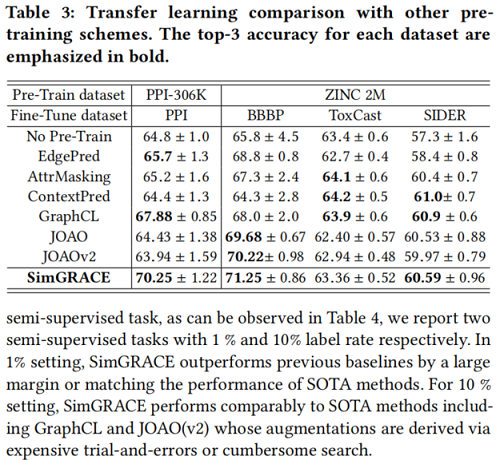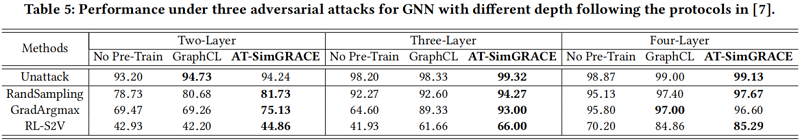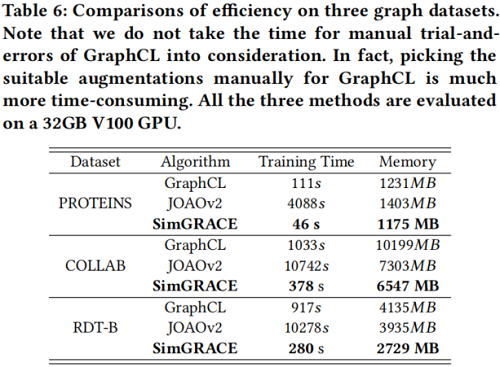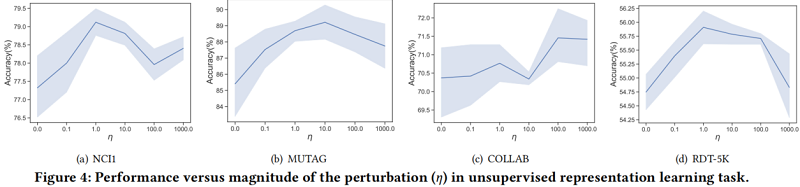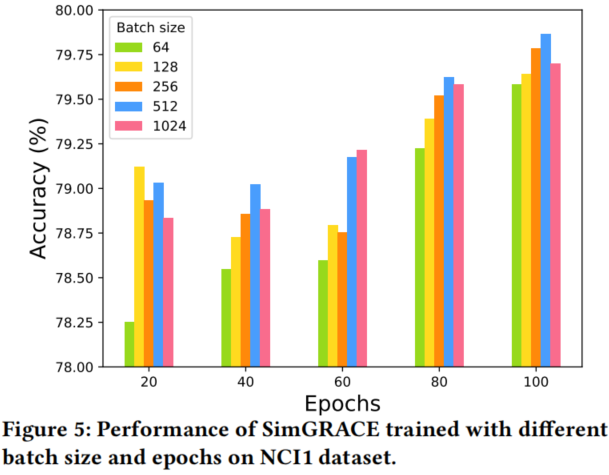# 4 Conclusions

在本文中，我们提出了一个简单的图对比学习框架(SimGRACE)。虽然它看起来很简单，但我们证明了SimGRACE可以在不同规模和类型的多个图形数据集上超越或匹配最先进的竞争对手，同时享有前所未有的灵活性、高效和易用性。我们将图对比学习从繁琐的手动调优、繁琐的搜索或昂贵的领域知识中解放出来。此外，我们还设计了对抗性训练方案来原则性地提高SimGRACE的鲁棒性，并从理论上解释了其原因。未来的工作有两个很有前途的途径：（1）探索编码器扰动是否可以在计算机视觉和自然语言处理等其他领域的工作良好。（2）将预先训练过的gnn应用于更现实世界的任务，包括社会分析和生物化学。

# References

Graph Contrastive Learning Automated (ICML 2021)
Graph Contrastive Learning with Augmentations (NeurIPS 2020)
Strategies for Pre-training Graph Neural Networks (ICLR 2020)
Adversarial Attack on Graph Structured Data (ICML 2018)